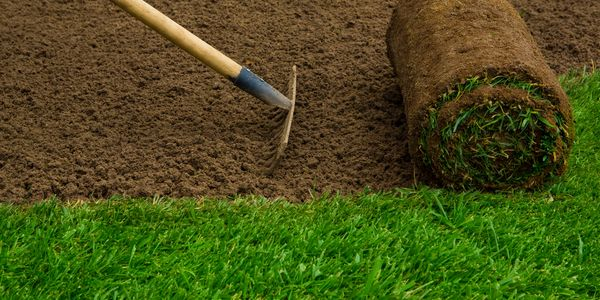260 Route 565 Wantage We Have added to our inventory! We now have mulch and stone.

#### What is a "yard" of material?A yard of topsoil, mulch or stone is 27 cubic feet of material: 3 feet wide by 3 feet long by 3 feet high

#### How do I calculate how much material I need?Step 1: Determine how thick you would like the coverage( ie. 2",3")

Step 2: Measure the area to be covered. Length times Width

Step 3: Divide footage from above by 27. The result will be for 1 foot thickness of material.

Step 4: For lighter coverage divide further

* for 6" divide total by 2

* for 4" divide total by 3

* for 3" divide total by 4

* for 2" divide total by 2

#### Example4" thick Mulch for a flower bed 18ft x 4ft

18ft x 4ft = 72 sq. ft.

72 sq. ft. / 27 = 2.6

2.6 / 3 =0.88 yards of material